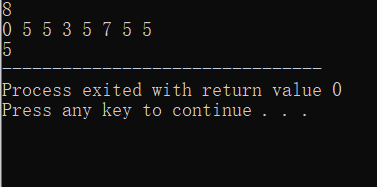# （此题重点！！！）已知一个整数序列 A =(a 0 ，a 1 ，..., a n-1 )，找出 A 的主元素

## 源代码

#include<iostream>
using namespace std;
int majority(int A[],int n)
{
int i,c,count=1;
c=A;
for(i=1;i<n;++i){
if(A[i]==c)
count++;
else{
if(count>0)
count--;
else{
c=A[i];
count=1;
}
}
}
if(count>0){
for(i=count=0;i<n;++i){
if(A[i]==c);
count++;
}
}
if(count>n/2)
return c;
else
return -1;
}

int main()
{
int n;
cin>>n;
int A1[n];
for(int i=0;i<n;++i){
cin>>A1[i];
}
cout<<majority(A1,n);
return 0;
}



## 执行结果©️2019 CSDN 皮肤主题: 1024 设计师: 上身试试